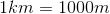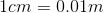# High School Physics : Understanding SI Units

## Example Questions

### Example Question #11 : Understanding Si Units

Which of these is an SI unit?

Acre

Joule

Fathom

Yard

Inch

Joule

Explanation:

Joules are the SI unit for energy.

Fathoms, yards, and inches are all measures of length, but the SI units for length are meters. Acres are a measure of area, but the SI unit of area would be meters-squared.

### Example Question #12 : Understanding Si Units

Which of these is not an SI unit?

Coulomb

Newton

Schrödinger

Hertz

Schrödinger

Explanation:

While Erwin Schrödinger was a physicist, there is currently no unit named after him.

Newtons are the SI unit for force, Farads are the SI unit for capacitance, Coulombs are the SI unit for charge, and hertz are the SI unit for frequency.

### Example Question #13 : Understanding Si Units

Which of these is not an SI unit?

Ampere

Watt

Ohm

Degree Celsius

Degree Celsius

Explanation:

Degrees Celsius is a measure of temperature, but the SI unit for temperature is Kelvin.

Amperes are the SI unit for current, Ohms are the SI unit for resistance, Farads are the SI unit for capacitance, and watts are the SI unit for power.

### Example Question #14 : Understanding Si Units

Which of these is not an SI unit?

Joule

Henry

Heisenberg

Ohm

Watt

Heisenberg

Explanation:

While Heisenberg is a famous chemist and physicist, no units have been named after him.

Watts are the SI units for power, Joules are the SI unit for energy, Ohms are the SI unit for resistance, and Henrys are the SI unit for inductance.

### Example Question #11 : Understanding Si Units

Which of the following is not an SI unit?

Kilogram

Newton-meter

Second

Vector

Vector

Explanation:

A vector is not a unit of measurement. A vector is a quantity with both magnitude and direction. This is different from a scalar, which has only magnitude but no direction. All units of measurement can be classified as either vectors or scalars. For example, velocity is a vector and speed is a scalar.

Kilograms are the SI units for mass. Farads are the SI unit for capacitance. Newton-meters are a compound SI unit that measure torque, and are equivalent to Joules. Seconds are the SI unit for time.

### Example Question #16 : Understanding Si Units

Which of these is not an SI unit?

Volt

Meter

Minute

Ohm

Joule

Minute

Explanation:

While a minute is an accurate measurement of time, the official SI unit for time is the second. That's why velocity is given in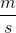and acceleration is given in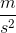.

Meters are the SI unit for length or distance. Joules are the SI unit for work or energy. Volts are the SI unit for electric potential. Ohms are the SI unit for electrical resistance.

### Example Question #12 : Understanding Si Units

Which of these is the correct SI unit for power?

Joule

Coulomb

Lumens

Horsepower

Watt

Watt

Explanation:

The correct SI unit for power is the Watt, which is equivalent to Joules per second. Joules are used to measure energy or work.

Coulombs are the SI unit for electrical charge and lumens are the SI units for brightness. Horsepower is a non-SI unit for power.

### Example Question #18 : Understanding Si Units

Miles per hour is a measure of __________. The SI unit for this measurement is __________.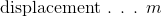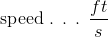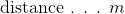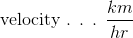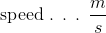Explanation:

All physics calculations require the use of proper standard units, known as SI units. "Miles per hour" is a measure of length (miles) per unit time (hour). A change in position per unit time can correspond to either speed or velocity. Velocity is the vector conversion of speed, giving a magnitude of speed in an applied direction.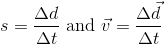The standard unit for distance or displacement is a meter and the standard unit for time is a second. In these equations, we can see that an answer will have the units "meters per second" once the distance is divided by the time.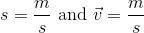Kilometers per hour and feet per second can also be used to measure a speed of velocity, but do not correspond to standard SI units.

### Example Question #19 : Understanding Si Units

What are the SI units for temperature?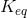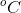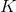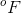Explanation:

All physics calculations require the use of proper standard units, known as SI units. When working with temperature, physics calculations require the use of the absolute temperature, given in the units of Kelvin. Kelvin use absolute zero as their initial reference, whereas degrees Celsius use the freezing point of water. Since absolute zero is equal to approximately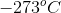, the conversion from Celsius to Kelvin is simply to add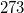.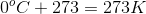Degrees Fahrenheit are a relatively non-standard unit for measuring temperature, and require a more complicated conversion.refers to the equilibrium constant for chemical reactions. Though this value is dependent on temperature, it is not a unit of measurement and is not used in physics calculations.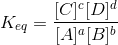can refer to a few different things in physics, but is never used as a unit of measurement. This variable generally refers to Coulomb's constant in calculations with electric fields, but can also be used for the dielectric constant of a material or Boltzmann's constant.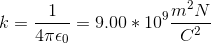### Example Question #20 : Understanding Si Units

What are the SI units for length?

Displacement

Distance

Meters

Kilometers

Centimeters

Meters

Explanation:

All physics calculations require the use of proper standard units, known as SI units. It is important to convert all terms to SI units before proceeding to use any formulae.

Distance and displacement are quantifications of length. The SI unit for length is meters; thus any calculation requiring a distance or displacement term must use meters for this variable.

One kilometer is equivalent to one thousand meters. One hundred centimeters are equivalent to one meter.